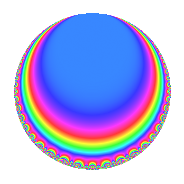# Properties

 Label 600.2.f.eLevel 600 Weight 2 Character orbit 600.f Analytic conductor 4.791 Analytic rank 0 Dimension 2 CM No Inner twists 2

# Learn more about

## Newspace parameters

 Level: $$N$$ = $$600 = 2^{3} \cdot 3 \cdot 5^{2}$$ Weight: $$k$$ = $$2$$ Character orbit: $$[\chi]$$ = 600.f (of order $$2$$ and degree $$1$$)

## Newform invariants

 Self dual: No Analytic conductor: $$4.79102412128$$ Analytic rank: $$0$$ Dimension: $$2$$ Coefficient field: $$\Q(\sqrt{-1})$$ Coefficient ring: $$\Z[a_1, a_2, a_3]$$ Coefficient ring index: $$1$$ Sato-Tate group: $\mathrm{SU}(2)[C_{2}]$

## $q$-expansion

Coefficients of the $$q$$-expansion are expressed in terms of $$i = \sqrt{-1}$$. We also show the integral $$q$$-expansion of the trace form.

 $$f(q)$$ $$=$$ $$q$$ $$+ i q^{3}$$ $$- q^{9}$$ $$+O(q^{10})$$ $$q$$ $$+ i q^{3}$$ $$- q^{9}$$ $$+ 4 q^{11}$$ $$+ 2 i q^{13}$$ $$+ 2 i q^{17}$$ $$+ 4 q^{19}$$ $$+ 8 i q^{23}$$ $$-i q^{27}$$ $$-6 q^{29}$$ $$+ 8 q^{31}$$ $$+ 4 i q^{33}$$ $$+ 6 i q^{37}$$ $$-2 q^{39}$$ $$-6 q^{41}$$ $$-4 i q^{43}$$ $$+ 7 q^{49}$$ $$-2 q^{51}$$ $$+ 2 i q^{53}$$ $$+ 4 i q^{57}$$ $$-4 q^{59}$$ $$-2 q^{61}$$ $$-4 i q^{67}$$ $$-8 q^{69}$$ $$+ 8 q^{71}$$ $$-10 i q^{73}$$ $$+ 8 q^{79}$$ $$+ q^{81}$$ $$+ 4 i q^{83}$$ $$-6 i q^{87}$$ $$+ 6 q^{89}$$ $$+ 8 i q^{93}$$ $$+ 2 i q^{97}$$ $$-4 q^{99}$$ $$+O(q^{100})$$ $$\operatorname{Tr}(f)(q)$$ $$=$$ $$2q$$ $$\mathstrut -\mathstrut 2q^{9}$$ $$\mathstrut +\mathstrut O(q^{10})$$ $$2q$$ $$\mathstrut -\mathstrut 2q^{9}$$ $$\mathstrut +\mathstrut 8q^{11}$$ $$\mathstrut +\mathstrut 8q^{19}$$ $$\mathstrut -\mathstrut 12q^{29}$$ $$\mathstrut +\mathstrut 16q^{31}$$ $$\mathstrut -\mathstrut 4q^{39}$$ $$\mathstrut -\mathstrut 12q^{41}$$ $$\mathstrut +\mathstrut 14q^{49}$$ $$\mathstrut -\mathstrut 4q^{51}$$ $$\mathstrut -\mathstrut 8q^{59}$$ $$\mathstrut -\mathstrut 4q^{61}$$ $$\mathstrut -\mathstrut 16q^{69}$$ $$\mathstrut +\mathstrut 16q^{71}$$ $$\mathstrut +\mathstrut 16q^{79}$$ $$\mathstrut +\mathstrut 2q^{81}$$ $$\mathstrut +\mathstrut 12q^{89}$$ $$\mathstrut -\mathstrut 8q^{99}$$ $$\mathstrut +\mathstrut O(q^{100})$$

## Character Values

We give the values of $$\chi$$ on generators for $$\left(\mathbb{Z}/600\mathbb{Z}\right)^\times$$.

 $$n$$ $$151$$ $$301$$ $$401$$ $$577$$ $$\chi(n)$$ $$1$$ $$1$$ $$1$$ $$-1$$

## Embeddings

For each embedding $$\iota_m$$ of the coefficient field, the values $$\iota_m(a_n)$$ are shown below.

For more information on an embedded modular form you can click on its label.

Label $$\iota_m(\nu)$$ $$a_{2}$$ $$a_{3}$$ $$a_{4}$$ $$a_{5}$$ $$a_{6}$$ $$a_{7}$$ $$a_{8}$$ $$a_{9}$$ $$a_{10}$$
49.1
 − 1.00000i 1.00000i
0 1.00000i 0 0 0 0 0 −1.00000 0
49.2 0 1.00000i 0 0 0 0 0 −1.00000 0
 $$n$$: e.g. 2-40 or 990-1000 Significant digits: Format: Complex embeddings Normalized embeddings Satake parameters Satake angles

## Inner twists

Char. orbit Parity Mult. Self Twist Proved
1.a Even 1 trivial yes
5.b Even 1 yes

## Hecke kernels

This newform can be constructed as the intersection of the kernels of the following linear operators acting on $$S_{2}^{\mathrm{new}}(600, [\chi])$$:

 $$T_{7}$$ $$T_{11}$$ $$\mathstrut -\mathstrut 4$$